# Free Printable 2 Multiplication Table Charts in PDF

2 Multiplication Table Charts: When the child promoted to Grade 1, the very first thing a teacher makes them learn is multiplication tables. Then and their thought strike to their mind what does this mean? What is the teacher talking about? Their mind is full of questions just because the teacher told them today, we will learn multiplication tables.

Multiplication Table is a list of a particular number where one number is along a row and another down a column, and the result is shown where row and column meet. For instance, 2 multiplied by 9 is 18. Our article is about the 2 Multiplication Table, which is considered as a primary table for every child in the school.

It is very easy to learn in the grid format or complete format such as 2*2=4. Table of 2 you can memorize by adding only number 02 to any other even number and how many times you are adding likewise if you add number 02 to number 04 you will get 06. You add number 02 3 times i.e. 2+2+2=6

In this article, we have come up with different formats of 2 Multiplication Table where your child is able to learn very easily include worksheets for practice, chart, and learning while playing.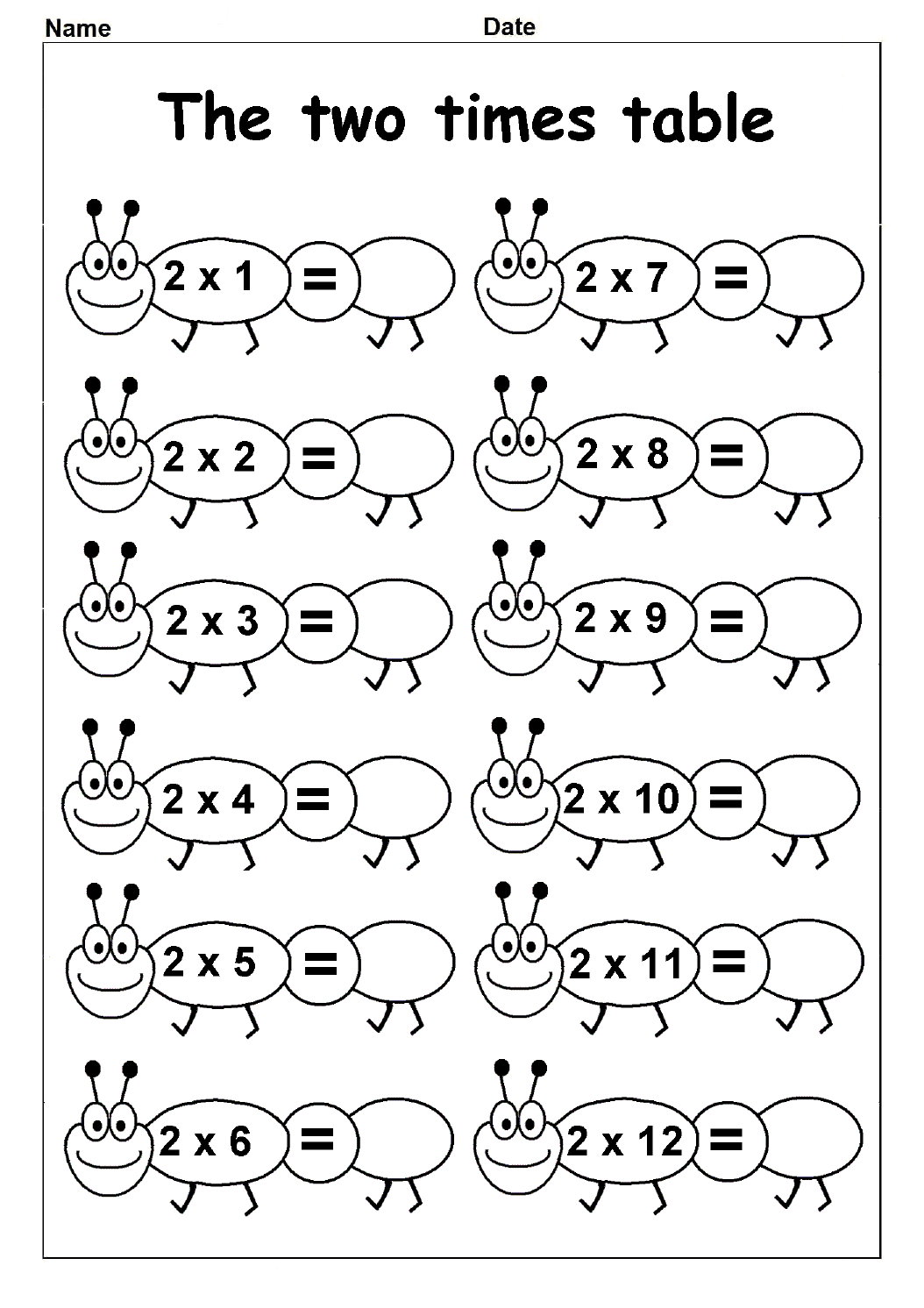PDF

## Multiplication Table of 2 Worksheet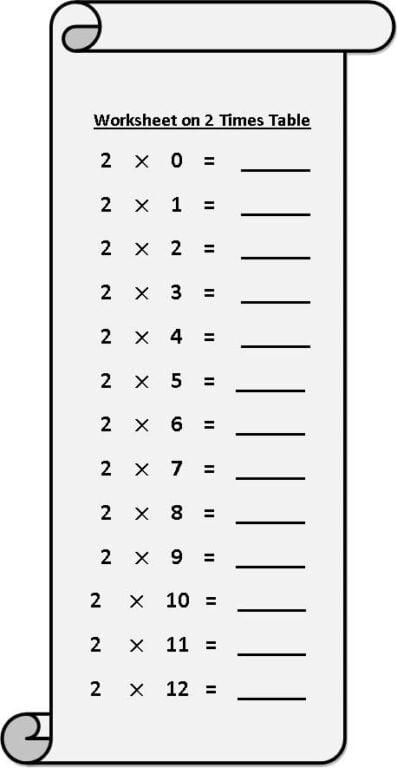PDF

You can download free practice worksheet from here in pdf format which means portable documented file, the most reliable files, then take print of that as many as you required as per your need. It is like learning an online reading and math program. This worksheet made accessible for testing their knowledge and based on finding the product. We have created colorful and creative worksheets for the kids. “Learning while Playing”

## 2 Times Tables Chart

What does it mean 2 Multiplication Table chart? It means a table that shows you the consequences of the product of two numbers. One number is along a row, the other down a column, and the outcomes are indicated where a line and section meet.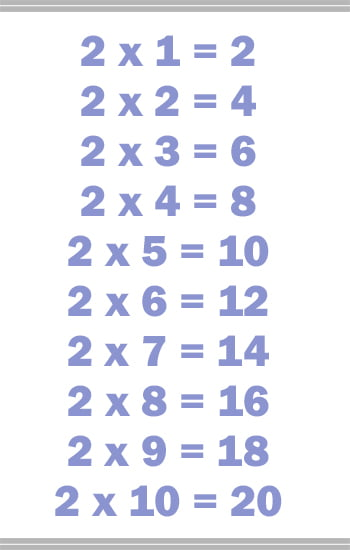PDF

My question to all is how do you read two times the table? Just give it a thought in your mind. Read below mention: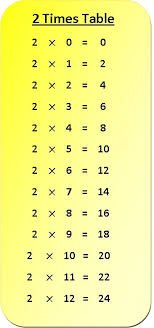PDF

We read two times the table as One time two is 2. Two times two is 4. Three times two is 6. Four times two is 8. Five times two is 10. Six times two is 12. Seven times two is 14. Eight times two is 16 and much more.

### 2 Times Table Games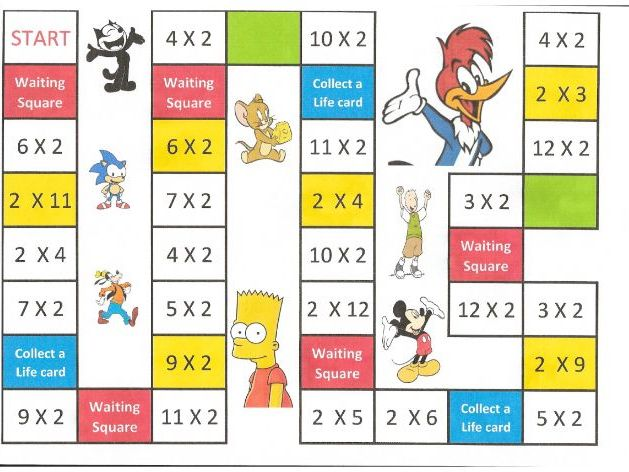PDF

Make your child learn tables through games or while playing as every school does for kids. We will provide you 2 Multiplication Table of different kinds of practice and children will learn in fun also.

Step 1b: In sequence

You can take print of the same and then fill in your answers.

Next game you can ask your child to choose the correct answers and then fill them. For this, you can download it in PDF format and get a print of it.

Here we have shuffled the table and you have to ask your child to fill them. With this little activity confidence of your child will boost up and most indispensable is to give them a chance to practice worksheet a lot. This will aid them to recall, memorize, and enable them for the quick multiplication with no pen or calculator.